Search by Topic

Resources tagged with Resourceful similar to Attractive Tablecloths:

Filter by: Content type:
Age range:
Challenge level:

There are 59 results

Broad Topics > Habits of Mind > ResourcefulAttractive Tablecloths

Age 14 to 16 Challenge Level:

Charlie likes tablecloths that use as many colours as possible, but insists that his tablecloths have some symmetry. Can you work out how many colours he needs for different tablecloth designs?Frogs

Age 11 to 14 Challenge Level:

How many moves does it take to swap over some red and blue frogs? Do you have a method?Marbles in a Box

Age 11 to 16 Challenge Level:

How many winning lines can you make in a three-dimensional version of noughts and crosses?Age 11 to 14 Challenge Level:

How many different symmetrical shapes can you make by shading triangles or squares?Tower of Hanoi

Age 11 to 14 Challenge Level:

The Tower of Hanoi is an ancient mathematical challenge. Working on the building blocks may help you to explain the patterns you notice.Reversals

Age 11 to 14 Challenge Level:

Where should you start, if you want to finish back where you started?Special Numbers

Age 11 to 14 Challenge Level:

My two digit number is special because adding the sum of its digits to the product of its digits gives me my original number. What could my number be?On the Edge

Age 11 to 14 Challenge Level:

If you move the tiles around, can you make squares with different coloured edges?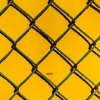Finding Factors

Age 14 to 16 Challenge Level:

Can you find the hidden factors which multiply together to produce each quadratic expression?Multiplication Arithmagons

Age 14 to 16 Challenge Level:

Can you find the values at the vertices when you know the values on the edges of these multiplication arithmagons?How Much Can We Spend?

Age 11 to 14 Challenge Level:

A country has decided to have just two different coins, 3z and 5z coins. Which totals can be made? Is there a largest total that cannot be made? How do you know?Arithmagons

Age 14 to 16 Challenge Level:

Can you find the values at the vertices when you know the values on the edges?Semi-regular Tessellations

Age 11 to 16 Challenge Level:

Semi-regular tessellations combine two or more different regular polygons to fill the plane. Can you find all the semi-regular tessellations?How Old Am I?

Age 14 to 16 Challenge Level:

In 15 years' time my age will be the square of my age 15 years ago. Can you work out my age, and when I had other special birthdays?A Little Light Thinking

Age 14 to 16 Challenge Level:

Here is a machine with four coloured lights. Can you make two lights switch on at once? Three lights? All four lights?Which Solids Can We Make?

Age 11 to 14 Challenge Level:

Interior angles can help us to work out which polygons will tessellate. Can we use similar ideas to predict which polygons combine to create semi-regular solids?Age 11 to 14 Challenge Level:

What happens when you add a three digit number to its reverse?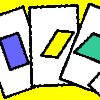Age 11 to 14 Challenge Level:

A game for 2 or more people, based on the traditional card game Rummy. Players aim to make two `tricks', where each trick has to consist of a picture of a shape, a name that describes that shape, and. . . .Square It

Age 11 to 16 Challenge Level:

Players take it in turns to choose a dot on the grid. The winner is the first to have four dots that can be joined to form a square.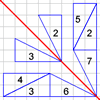Transformation Game

Age 11 to 14 Challenge Level:

Why not challenge a friend to play this transformation game?Isosceles Triangles

Age 11 to 14 Challenge Level:

Draw some isosceles triangles with an area of $9$cm$^2$ and a vertex at (20,20). If all the vertices must have whole number coordinates, how many is it possible to draw?Cryptarithms

Age 11 to 14 Challenge Level:

Can you crack these cryptarithms?Unequal Averages

Age 11 to 14 Challenge Level:

Play around with sets of five numbers and see what you can discover about different types of average...A Chance to Win?

Age 11 to 14 Challenge Level:

Imagine you were given the chance to win some money... and imagine you had nothing to lose...Where Can We Visit?

Age 11 to 14 Challenge Level:

Charlie and Abi put a counter on 42. They wondered if they could visit all the other numbers on their 1-100 board, moving the counter using just these two operations: x2 and -5. What do you think?Stars

Age 11 to 14 Challenge Level:

Can you find a relationship between the number of dots on the circle and the number of steps that will ensure that all points are hit?Two's Company

Age 11 to 14 Challenge Level:

7 balls are shaken in a container. You win if the two blue balls touch. What is the probability of winning?Cosy Corner

Age 11 to 14 Challenge Level:

Six balls of various colours are randomly shaken into a trianglular arrangement. What is the probability of having at least one red in the corner?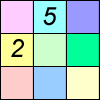Difference Sudoku

Age 14 to 16 Challenge Level:

Use the differences to find the solution to this Sudoku.Constructing Triangles

Age 11 to 14 Challenge Level:

Generate three random numbers to determine the side lengths of a triangle. What triangles can you draw?Cuboids

Age 11 to 14 Challenge Level:

Find a cuboid (with edges of integer values) that has a surface area of exactly 100 square units. Is there more than one? Can you find them all?Substitution Cipher

Age 11 to 14 Challenge Level:

Find the frequency distribution for ordinary English, and use it to help you crack the code.Treasure Hunt

Age 7 to 14 Challenge Level:

Can you find a reliable strategy for choosing coordinates that will locate the treasure in the minimum number of guesses?Which Spinners?

Age 14 to 18 Challenge Level:

Can you work out which spinners were used to generate the frequency charts?Gabriel's Problem

Age 11 to 14 Challenge Level:

Gabriel multiplied together some numbers and then erased them. Can you figure out where each number was?Property Chart

Age 11 to 14 Challenge Level:

A game in which players take it in turns to try to draw quadrilaterals (or triangles) with particular properties. Is it possible to fill the game grid?Hexy-metry

Age 14 to 16 Challenge Level:

A hexagon, with sides alternately a and b units in length, is inscribed in a circle. How big is the radius of the circle?Triangles to Tetrahedra

Age 11 to 14 Challenge Level:

Imagine you have an unlimited number of four types of triangle. How many different tetrahedra can you make?Shapely Pairs

Age 11 to 14 Challenge Level:

A game in which players take it in turns to turn up two cards. If they can draw a triangle which satisfies both properties they win the pair of cards. And a few challenging questions to follow...Charlie's Delightful Machine

Age 11 to 16 Challenge Level:

Here is a machine with four coloured lights. Can you develop a strategy to work out the rules controlling each light?Shifting Times Tables

Age 11 to 14 Challenge Level:

Can you find a way to identify times tables after they have been shifted up or down?Two and Two

Age 11 to 14 Challenge Level:

How many solutions can you find to this sum? Each of the different letters stands for a different number.Nice or Nasty

Age 7 to 14 Challenge Level:

There are nasty versions of this dice game but we'll start with the nice ones...Funny Factorisation

Age 11 to 14 Challenge Level:

Using the digits 1 to 9, the number 4396 can be written as the product of two numbers. Can you find the factors?The Spider and the Fly

Age 14 to 16 Challenge Level:

A spider is sitting in the middle of one of the smallest walls in a room and a fly is resting beside the window. What is the shortest distance the spider would have to crawl to catch the fly?Dozens

Age 7 to 14 Challenge Level:

Do you know a quick way to check if a number is a multiple of two? How about three, four or six?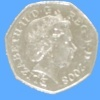Last One Standing

Age 14 to 16 Challenge Level:

Imagine a room full of people who keep flipping coins until they get a tail. Will anyone get six heads in a row?Product Sudoku

Age 11 to 14 Challenge Level:

The clues for this Sudoku are the product of the numbers in adjacent squares.In a Box

Age 14 to 16 Challenge Level:

Chris and Jo put two red and four blue ribbons in a box. They each pick a ribbon from the box without looking. Jo wins if the two ribbons are the same colour. Is the game fair?Odds and Evens

Age 11 to 14 Challenge Level:

Is this a fair game? How many ways are there of creating a fair game by adding odd and even numbers?